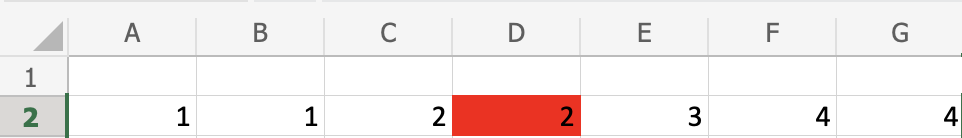# Excel MEDIAN Function

## MEDIAN Function

The MEDIAN function is a premade function in Excel, which returns the middle value in the data.

It is typed `=MEDIAN`

Note: The median is a type of average value, which describes where the center of the data is located. You can learn about median in our Statistics Median Tutorial.

Calculating the median manually requires that the data is sorted and arranged from low to high. This is not necessary using the MEDIAN function, it does it for you.

How to use the `=MEDIAN` function, step by step:

1. Select a cell (`H2`)
2. Type `=MEDIAN`
3. Double click the MEDIAN command
4. Select a range (`A2:G2`)
5. Hit enter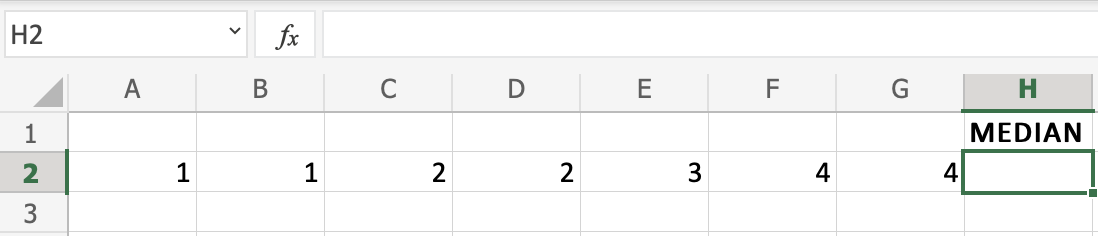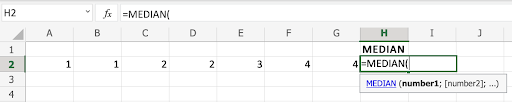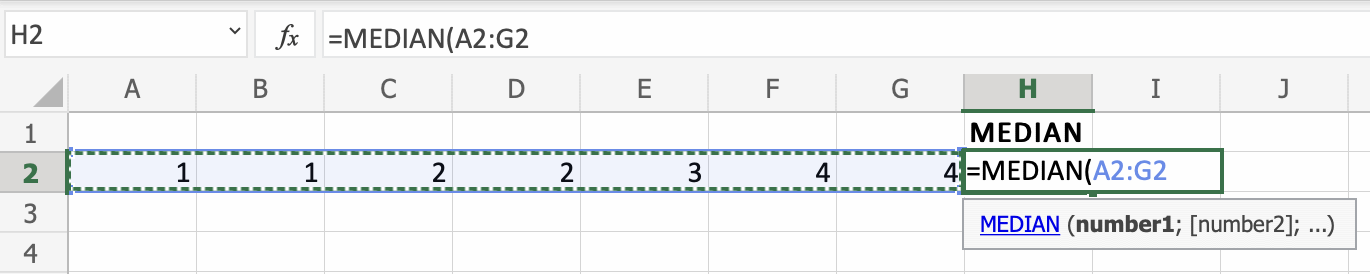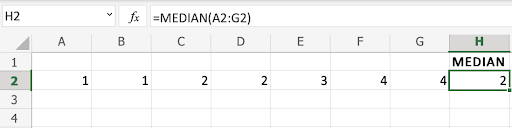Great! You successfully found the median using the `=MEDIAN` function.

Note that it found the value in the middle of the data, which is `D2(2)`, the one that is marked red: